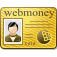# Control computer workshop RFEI Mathematics 30 z

Replenishment date: 14.06.2013
Content: matematika_kontr_komp_praktikum_30_z.rar (616.24 KB)
️Automatic issue of goods ✔️
Sales:
6
Refunds:
0
Reviews:
0
Views:
264SellerSeller:
GoodChecking
Rating:
0
Report a violation
Seller discounts
for all goods
The goods are given a discount for regular customers.
If the total purchases from a GoodChecking seller are greater than:
the discount is: 3%
Description
Control computer practice Mathematics 30 tasks.
Answers to 30 tasks are given, in the form of a table.
All tasks are contained in a separate file.

1. Calculate the determinant by expanding the elements of the third column:
2. Calculate the determinant by expanding the elements of the first line:
3. Calculate the determinant by expanding the elements of the first column:
4. Calculate the determinant by expanding the elements of the fourth line:
5. Calculate the determinant by expanding the elements of the third line:
6. Find the product of matrices A • B manually and check the result in MS Excel:
7. Find the product of matrices A • B manually and check the result in MS Excel:
8. Find the product of matrices in MS Excel.
9. Find the product of matrices in MS Excel.
10. Find the product of matrices in MS Excel.
11. Find the rank of the matrix.
12. Find the rank of the matrix.
13. Find the rank of the matrix.
14. Find the inverse of matrix A by choosing Fractional format (simple fractions):

...

27. Solve the system of linear equations using Cramer's formulas:
28. Solve the system of linear equations using Cramer's formulas:
30. Find the matrix ...